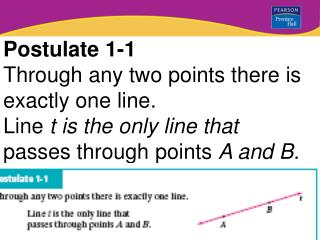DownloadDownload PresentationPostulate 1-1 Through any two points there is exactly one line. Line t is the only line that

# Postulate 1-1 Through any two points there is exactly one line. Line t is the only line that

Download Presentation## Postulate 1-1 Through any two points there is exactly one line. Line t is the only line that

- - - - - - - - - - - - - - - - - - - - - - - - - - - E N D - - - - - - - - - - - - - - - - - - - - - - - - - - -
##### Presentation Transcript

1. Postulate 1-1 Through any two points there is exactly one line. Line t is the only line that passes through points A and B.

2. Postulate 1-2 If two lines intersect, then they intersect in exactly one point. AE and BD intersect at C.

3. Postulate 1-3 If two planes intersect, then they intersect in exactly one line. Plane RST and plane STW intersect in ST.

4. Points, Lines, and Planes LESSON 1-3 Additional Examples In the figure below, name three points that are collinear and three points that are not collinear. Points Y, Z, and W lie on a line, so they are collinear. Any other set of three points do not lie on a line, so no other set of three points is collinear. For example, X, Y, and Z and X, W, and Z form triangles and are not collinear. Quick Check

5. Postulate 1-4 Through any three non-collinear points there is exactly one plane.

6. Points, Lines, and Planes LESSON 1-3 Additional Examples Name the plane shown in two different ways. You can name a plane using any three or more points on that plane that are not collinear. Some possible names for the plane shown are the following: plane RST plane RSU plane RTU plane STU Quick Check plane RSTU

7. The back and left faces of the cube intersect at HD. Planes HGC and AED intersect vertically at HD. Points, Lines, and Planes LESSON 1-3 Additional Examples Use the diagram below. What is the intersection of plane HGC and plane AED? As you look at the cube, the front face is on plane AEFB, the back face is on plane HGC, and the left face is on plane AED. Quick Check

8. Points X, Y, and Z are the vertices of one of the four triangular faces of the pyramid. To shade the plane, shade the interior of the triangle formed by X, Y, and Z. Points, Lines, and Planes LESSON 1-3 Additional Examples Shade the plane that contains X, Y, and Z. Quick Check